# Colleges with the highest SAT scores in Kentucky

Top 10 colleges in Kentucky with the highest SAT scores
Looking for the colleges with the highest SAT scores in Kentucky? Well you're in luck! We've compiled a national college database and have created a list of the top 10 universities with the highest SAT scores in Kentucky. These are the schools whose applicants had the highest average SAT scores in Kentucky. And, since these tests are meant to determine academic prowess, they are arguably the schools with the most academically proficient students. You could even say these are the best colleges in Kentucky. We also include each college's ACT scores and acceptance rate so that you can see where you would have the easiest or hardest time getting in. Read on to find out more.

## Centre College SAT scores

The average SAT score for Centre College is 1300.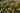The average SAT score of 1300 breaks down into:

• SAT math: 660

The average ACT score for Centre College is 29 and their acceptance rate is 76.3%.

## The University of Louisville SAT scores

The average SAT score for The University of Louisville is 1190.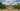The average SAT score of 1190 breaks down into:

• SAT math: 590

The average ACT score for The University of Louisville is 26 and their acceptance rate is 72.9%.

## University of Kentucky SAT scores

The average SAT score for University of Kentucky is 1190.The average SAT score of 1190 breaks down into:

• SAT math: 590

The average ACT score for University of Kentucky is 26 and their acceptance rate is 94.5%.

## Bellarmine University SAT scores

The average SAT score for Bellarmine University is 1170.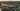The average SAT score of 1170 breaks down into:

• SAT math: 580

The average ACT score for Bellarmine University is 25 and their acceptance rate is 85.8%.

## Murray State University SAT scores

The average SAT score for Murray State University is 1150.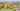The average SAT score of 1150 breaks down into:

• SAT math: 580

The average ACT score for Murray State University is 24 and their acceptance rate is 81.4%.

## Sullivan University SAT scores

The average SAT score for Sullivan University is 1140.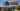The average SAT score of 1140 breaks down into:

• SAT math: 550

The average ACT score for Sullivan University is 21 and their acceptance rate is 84.1%.

## Asbury University SAT scores

The average SAT score for Asbury University is 1135.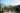The average SAT score of 1135 breaks down into:

• SAT math: 550

The average ACT score for Asbury University is 25 and their acceptance rate is 70.3%.

## Berea College SAT scores

The average SAT score for Berea College is 1123.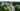The average SAT score of 1123 breaks down into:

• SAT math: 570

The average ACT score for Berea College is 25 and their acceptance rate is 38.2%.

## Baker University SAT scores

The average SAT score for Baker University is 1110.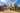The average SAT score of 1110 breaks down into:

• SAT math: 570

The average ACT score for Baker University is 23 and their acceptance rate is 67.3%.

## Northern Kentucky University SAT scores

The average SAT score for Northern Kentucky University is 1110.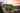The average SAT score of 1110 breaks down into:

• SAT math: 550